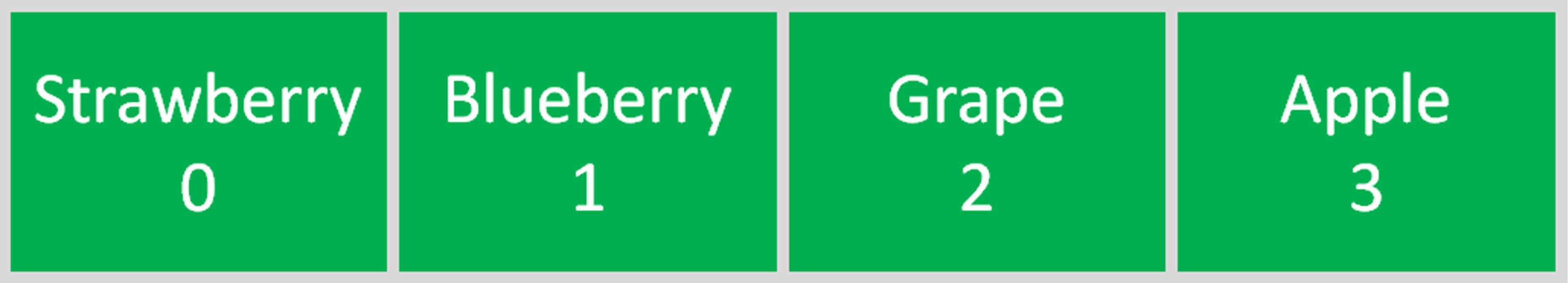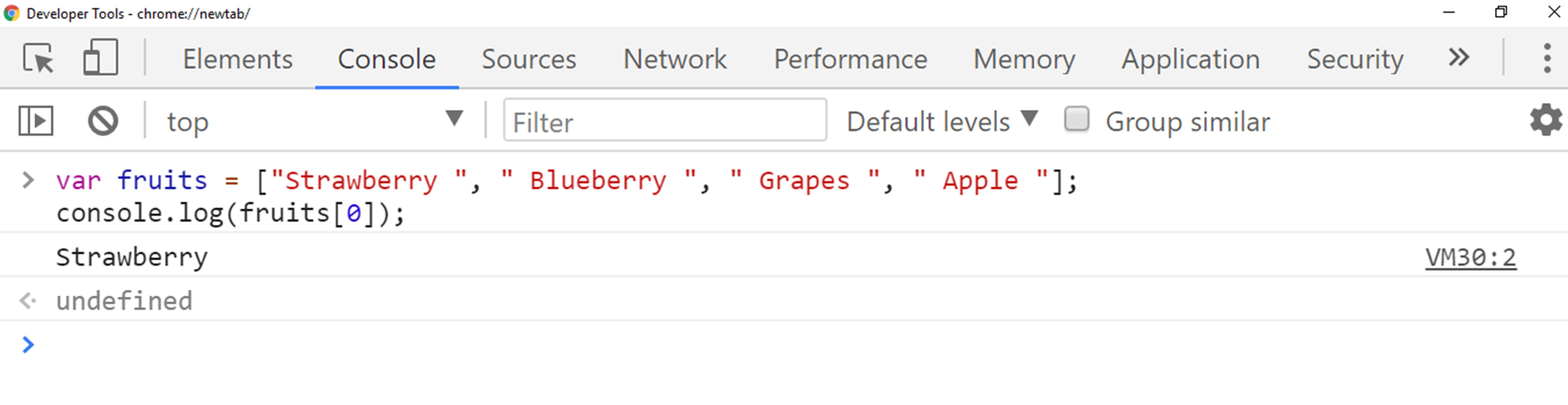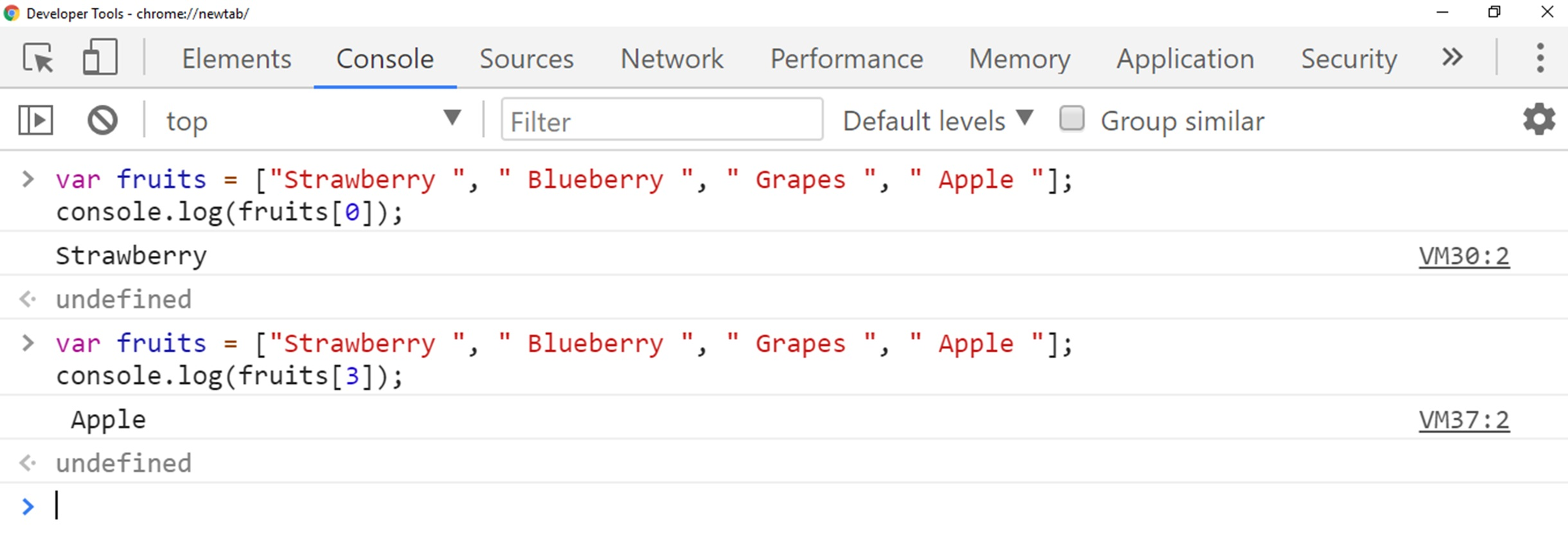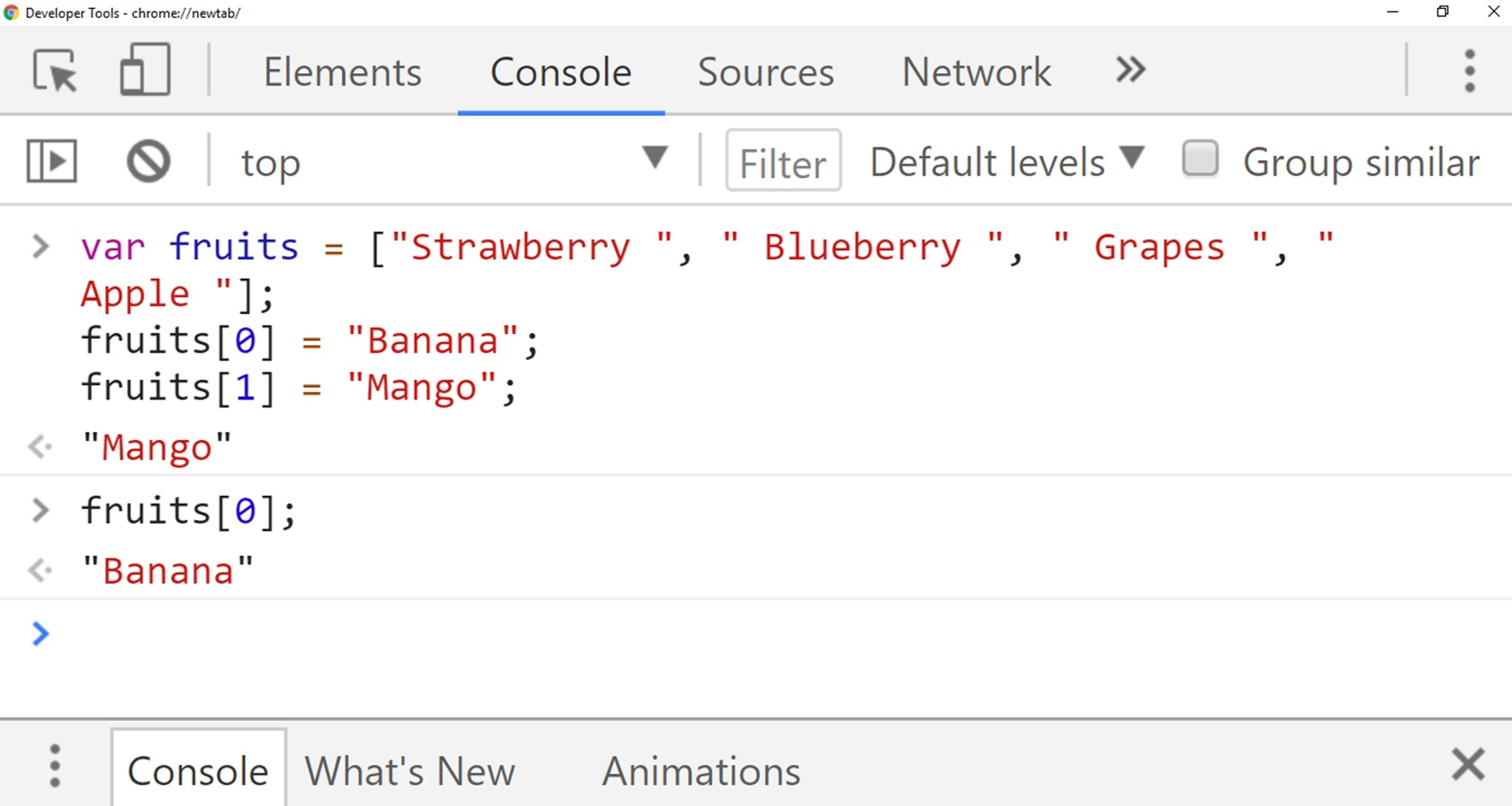# Lesson 16 Arrays In JavaScript

In this lesson, we are going to introduce array in JavaScript and we are going to learn how we can utilize it.
So in this lesson, we will discuss our first main data structure which is something called the array.
We will learn how we can code using arrays.

## Introduction to Arrays

The first one is we want to understand what arrays are and why we use them.
And then secondly, we want to use them in our code.
So let’s start by talking about conceptually what the point of an array is.

## Why we use Arrays

So let’s suppose I want to model a bunch of fruits, so every fruit is a string with their names like Strawberry, Blueberry, Grapes, and Apple.
So I could make four fruits variables, each one is a separate variable and if wanted to add another one I need to declare another variable.

``````var fruit1 = " Strawberry ";
var fruit2 = " Blueberry ";
var fruit3 = " Grapes";
var fruit4 = " Apple";
``````

So these fruits are not related to each other, they are totally separate and this is a lot repeated code.
So this code we can’t consider DRY. So this is so problematic.

### What is array

So this is a perfect use case for an array, an array let us put group data into a list.
So rather than having four separate variables, we can write one variable and inside of it, we store four different fruits.
So let’s take a closer look at how arrays work.
I have the same example code here.

``````var fruits = ["Strawberry ", " Blueberry ", " Grapes ", " Apple "];
``````

Var fruits equal and then square brackets [].
So that is what tells JavaScript this is an array.
So the square brackets denote an array.
You can see open bracket and closed bracket.
And inside we have our list of data to declare an array.
It’s a common separated list, so we have our values like Strawberry, Blueberry, Grapes, and Apple.
So it is called array with four item.

The second important thing about arrays is that they are indexed, so just like characters in a string where there is a specific number that corresponds to every character starting at zero.
Arrays are also indexed starting at zero.
So every slot in this array has a corresponding number.
When we make this array here with these four fruits.
Here is a diagram representing how the array is created in memory.
So we have Strawberry stored with the number 0 as an index, Strawberry with 1, Grapes with 2 and Apple with 3.## How we can initialize Array

So here we are initializing the same exact array.
And if I want to get Strawberry out of the array, I need to know the index where Strawberry stored which is 0.
So to retrieve strawberry we will write our variable name which is fruits followed by the square bracket and inside the square bracket, we will write index number where Strawberry located which is 0.

``````var fruits = ["Strawberry ", " Blueberry ", " Grapes ", " Apple "];
console.log(fruits);
``````

Here is output below for above array code:So fruits is going to give me Strawberry.
Likewise, if I want to retrieve apple we will write fruitsSo if I want to change strawberry to Banana and Blueberry with Mango so all I have to do is find the index of that fruits that I want to replace and set to new fruit name.
So then our arrays look like this where we have Banana at index 0, the same thing with Blueberry.So you can notice there we have Banana indexed there as a Banana.
So this is how we can replace values of arrays

## Conclusion

So In this lesson, we have discussed what arrays are and how we can use them in our code.
We have also learned how to initialize an array, store any value in an array list and how we can change the value of existing array.